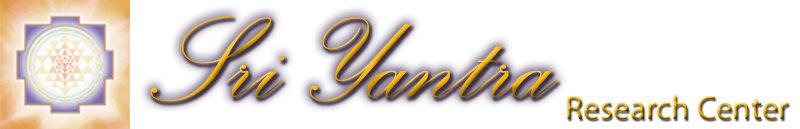The Spherical (Kurma) Sri Yantra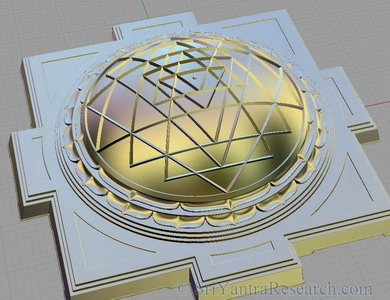Figure 1. Computer generated spherical Sri Yantra

 This form (as opposed to the plane or pyramid form) is particularly interesting because of it's higher degree of complexity and great beauty. The added complexity involved in creating a spherical Sri Yantra is probably why it is the rarest and why most of the one we have seen so far are poorly constructed. In this article we will look more closely at this fascinating configuration of the Sri Yantra and how the lines of this version can be straight and curved at the same time. So far we haven't been able to find a spherical Sri Yantra done properly except for the version that we have created with the help of a 3D drawing program and mathematical modelisation (figure 1). The reason is that the spherical version greatly increases the difficulty of achieving a correct figure. The added dimension increases the number of combinations exponentially. In other words it becomes a lot more difficult to drawn the figure properly when doing so on a dome rather than on a flat surface. The pyramid form is really an extended version of the plane version so it is not more difficult to produce than the plane form. You can see pictures of different version of the spherical Sri Yantra here. When we look closely we find that all but the computer generated version at the top are faulty. Lets take the copper figure in the image below as an example: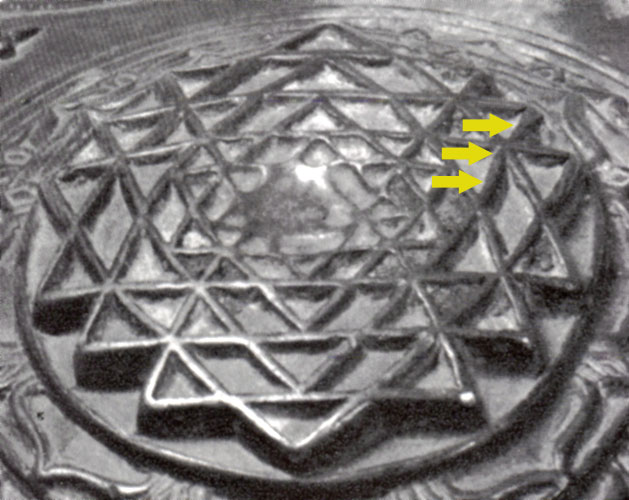Figure 2. Serious fudging can be seen on many of the lines.

 The picture above shows a copper Sri Yantra that is part of Ajit Mookerjee's private collection. At first look the figure appears correct but when we take a closer look we see that the artists wasn't able to make the lines match so a certain amount of cheating was necessary. The yellow arrows show how the artist had to bend this line to make it match at the triple intersection. If you look carefully you will notice that other lines had to be bent in the same way. Another important aspect of a spherical Sri Yantra is the specific curvature of the lines used in the figure. The curvatures of the lines comes from the fact that we are drawing triangles on a spherical surface. But the lines (when drawn properly) are actually straight lines drawn on the surface of a sphere. Like the path of an airplane flying from New York to Paris. If you look at the trajectory directly from above, the line will appear straight, but if we look at it from an angle it will look curved. The illustration below shows this phenomena with a properly constructed spherical Sri Yantra. The green line appears straight in the middle image when we look at it from straight above or in other words when our point of view is perpendicular to the line.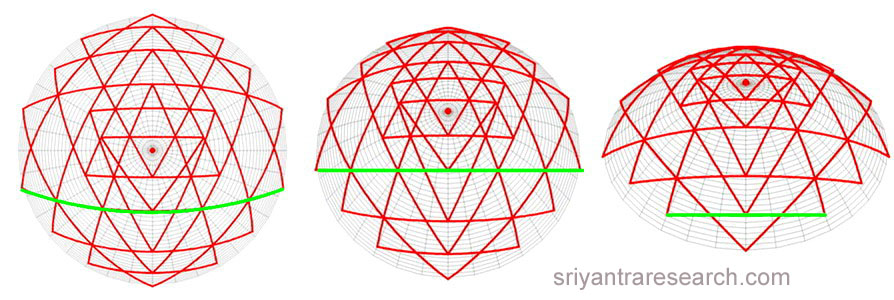Figure 3. The spherical Sri Yantra is constructed with straight lines on a sphere (arcs of great circles).

 Such a line is created on a sphere if it is a segment of a "great circle". A great circle is created on a sphere when a circle drawn on the surface has it's center located at the center of the sphere. For example, longitude lines around the planet are all great circles, latitude lines on the other hand are not except at the equator. This characteristic is very interesting. It means that if a spherical Sri Yantra is constructed with arcs of great circles (as it should) we really have straight lines on a sphere. So in a way we are preserving the qualities of the plane Sri Yantra and adding curvature in another axis, both coexisting at the same time. The spherical version therefore adds a new dimension but doesn't loose the basic qualities of the plane figure. When we look at the overall figure from above (figure 3, left figure) the lines appear more and more curved as we move away from the center. This is because our position is perpendicular to the center point only and because we are relatively close to the surface. If we move around we would see that every line is actually straight when we look at it from directly above. Also if we were to back off far enough the Sri Yantra would look identical to a plane Sri Yantra. Also if we choose to take a very small part of a sphere to draw the spherical form we will end up with a plane version. Because a very small area of a sphere is for all practical purpose flat. The very reason why the Earth was believed to be flat by many people for a long time. This shows that the plane version is not different from the spherical version but is a special case of the spherical form. A spherical Sri Yantra can be projected in 2D to obtain a plane figure with curved lines.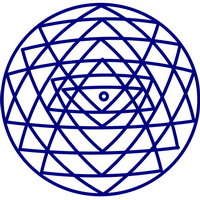figure 4. Spherical Sri Yantra projection in 2D.

 A proper spherical Sri Yantra must therefore be done with arcs of great circles and must obviously be at least concurrent (matching triple intersections). The computer generated drawing shown in figure 1 achieves those two criteria's as well as concentricity and equilateral central triangle as illustrated in the figure below. More information on these 3 criterias can be found here.Figure 5. The three criteria's of an optimal Sri Yantra.

 Going back to figure 2 we can see that the curvature of the lines are not proportional to the curvature of the dome. In other words the curvature of the lines doesn't match the curvature of the overall sculpture making it more of a projection in two dimensions rather than a true spherical Sri Yantra.